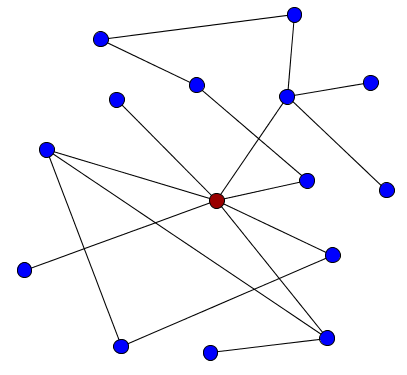# Math Insight

### Scale-free networks

A common feature of real world networks is the presence of hubs, or a few nodes that are highly connected to other nodes in the network. The presence of hubs will give the degree distribution a long tail, indicating the presence of nodes with a much higher degree than most other nodes.The red node is an example of a hub.

Scale-free networks are a type of network characterized by the presence of large hubs. A scale-free network is one with a power-law degree distribution. For an undirected network, we can just write the degree distribution as \begin{gather*} P_{\text{deg}}(k) \propto k^{-\gamma}, \end{gather*} where $\gamma$ is some exponent. This form of $P_{\text{deg}}(k)$ decays slowly as the degree $k$ increases, increasing the likelihood of finding a node with a very large degree.

Networks with power-law distributions a called scale-free1 because power laws have the same functional form at all scales. The power law $P_{\text{deg}}(k)$ remains unchanged (other than a multiplicative factor) when rescaling the independent variable $k$, as it satisfies $P_{\text{deg}}(ak)=a^{-\gamma} P_{\text{deg}}(k)$.

The above figure illustrates the degree distribution of a scale-free network of $N=10,000$ nodes and power-law exponent $\gamma=2$. The average degree is about 7, but 3/4 of the nodes have a degree of 3 or less. In the first bar plot, you cannot see that there are nodes with degree larger than 100, but plotting the bar heights with a logarithmic scale (second bar plot) reveals the long tail of the degree distribution. Although most nodes have a very small degree, there are a few nodes with a degree above 500. These presence of hubs that are orders of magnitude larger in degree than most nodes is a characteristic of power law networks.

One can recognize that a degree distribution has a power-law form by plotting it on a log-log scale. As shown in the above scatter plot, the points will tend to fall along a line. The line get pretty messy, though, for large degree, as there are few points to average out the noise. One could use larger bins at the larger degrees in order to make the graph turn out nicer.

To create the above plots, we didn't actually generate any networks (click image to see the Python program used to generate the figures). One way to generate scale-free networks is using a preferential attachment algorithm.1 If you add new nodes to a network and preferentially attach them to the nodes with high degrees, the “rich get richer” and you end up with hubs of very high degree.

Another way to generate scale-free networks is to use the models that generate networks with given degree distributions.